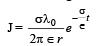Courses

# Single Correct MCQ Of Current Electricity, Past Year Questions JEE Advance, Class 12, Physics

## 33 Questions MCQ Test Class 12 Physics 35 Years JEE Mains &Advance Past year Paper | Single Correct MCQ Of Current Electricity, Past Year Questions JEE Advance, Class 12, Physics

Description
This mock test of Single Correct MCQ Of Current Electricity, Past Year Questions JEE Advance, Class 12, Physics for JEE helps you for every JEE entrance exam. This contains 33 Multiple Choice Questions for JEE Single Correct MCQ Of Current Electricity, Past Year Questions JEE Advance, Class 12, Physics (mcq) to study with solutions a complete question bank. The solved questions answers in this Single Correct MCQ Of Current Electricity, Past Year Questions JEE Advance, Class 12, Physics quiz give you a good mix of easy questions and tough questions. JEE students definitely take this Single Correct MCQ Of Current Electricity, Past Year Questions JEE Advance, Class 12, Physics exercise for a better result in the exam. You can find other Single Correct MCQ Of Current Electricity, Past Year Questions JEE Advance, Class 12, Physics extra questions, long questions & short questions for JEE on EduRev as well by searching above.
QUESTION: 1

### The temperature coefficient of resistance of a wire is 0.00125 per °C. At 300 K, its resistance is 1 ohm. This resistance of the wire will be 2 ohm at.

Solution: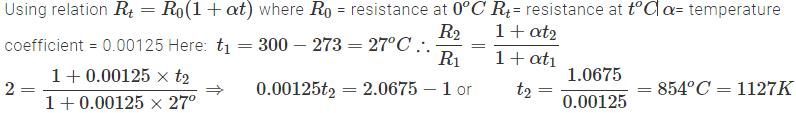QUESTION: 2

### A constant voltage is applied between the two ends of a uniform metallic wire. Some heat is developed in it. The heat developed is doubled if

Solution:

KEY CONCEPT : The heat produced is given by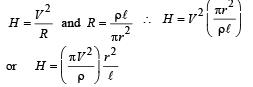Thus heat (H) is doubled if both length (l) and radius (r) are doubled.

QUESTION: 3

### The electrostatic field due to a point charge depends on the distance r as 1/r2 . Indicate which of the following quantities shows same dependence on r.

Solution: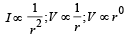QUESTION: 4

In the circuit shown in fig the heat produced in the 5 ohm resistor due to the current flowing through it is 10 calories per second.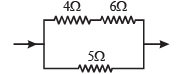The heat generated in the 4 ohms resistor is

Solution:

Since RAB = 2RCD therefore, current in AB will be half as compared to current in CD.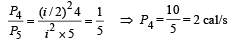Here P4 = Power dissipation in 4Ω

P5 = Power dissipation in 5Ω

QUESTION: 5

The current i in the circuit (see Fig) is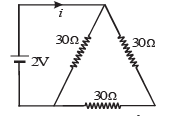Solution:

BC and AC are in series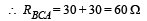Now BA and DC are in parallel.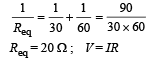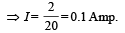QUESTION: 6

A piece of copper and another of germanium are cooled from room temperature to 80° K. The resistance of

Solution:

Copper is a metal whereas Germanium is Semi-conductor.
NOTE : Resistance of metal decreases and semiconductor increases with decrease in temperature.

QUESTION: 7

A battery of internal resistance 4Ω is connected to the network of resistances as shown. In order that the maximum power can be delivered to the network, the value of R in Ω should be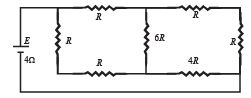Solution:

The equivalent circuits are shown in the figure.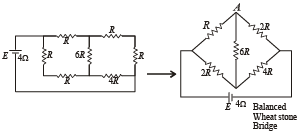The circuit represents balanced Wheatstone Bridge.
Hence 6R Ω resistance  is ineffective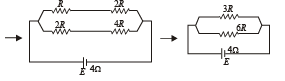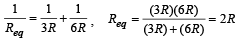For Max. Power External Resistance = Internal Resistance 2R = 4 Ω ∴ R = 2Ω

QUESTION: 8

In the  circuit P≠R , the reading of the galvanometer is same with switch S open or closed. Then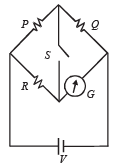Solution:

Since the opening or closing the switch does not affect the current through G, it means that in both the cases there is no current passing through S. Thus potential at A is equal to potential at B and it is the case of balanced wheatstone bridge..

IP = IQ and IR = IG

QUESTION: 9

In the given circuit, with steady current, the potential drop across the capacitor must be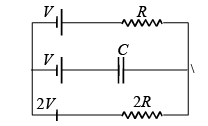Solution:

There will be no current flowing in branch BE in steady condition.
Let I be the current flowing in the loop ABCDEFA.
Applying Kirchoff's law in the loop moving in anticlockwise direction starting from C. + 2V – I (2R) – I (R) – V = 0
∴V = 3IR
⇒ I = V/3R ... (1)

Applying Kirchoff's law in the circuit ABEFA we get on moving  in anticlockwise direction starting from B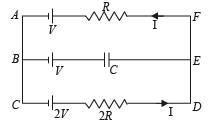+ V + Vcap – IR – V = 0 (where Vcap is the p.d. across capacitor).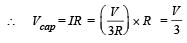QUESTION: 10

A wire of length L and 3 identical cells of negligible internal resistances are connected in series. Due to the current, the temperature of the wire is raised by ΔT in a time t. A number N of similar cells is now connected in series with a wire of the same material and cross-section but of length 2L. The temperature of the wire is raised by the same amount ΔT in the same time t. the value of N is

Solution:

Let R be the resistance of wire.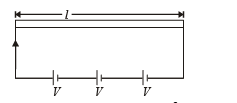Energy released in t second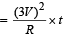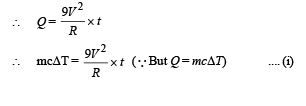Let R' be the resistance of the second wire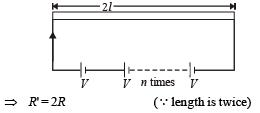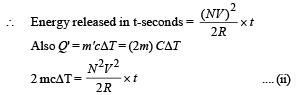Dividing (i) by (ii)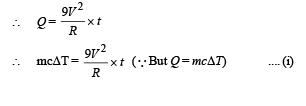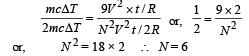or,        N 2 = 18 × 2    ∴ N = 6

QUESTION: 11

In the given circuit, it is observed that the current I is independent of the value of the resistance R6. Then the resistance values must satisfy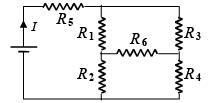Solution:

Since current I is independent of R6, it follows that the resistance R1, R2, R3 and R4 must form the balanced Wheatstone bridge.∴ R1 R4 = R2R3

QUESTION: 12

The effective resistance between points P and Q of the electrical circuit shown in the figure is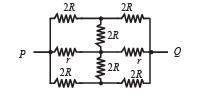Solution:

The circuit is symmetrical about the axis POQ.
The circuit above the axis POQ represents balanced wheatstone bride. Hence the central resistance 2R is ineffective. Similarly in the lower part (below the axis POQ) the central resistance 2R is ineffective.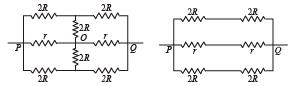Therefore the equivalent circuit is drawn.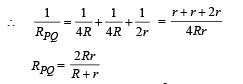QUESTION: 13

A 100 W bulb B1, and two 60 W bulb B2 an d B3, are connected to a 250 V source, as shown in figure. Now W1, W2 and W3 are the output powers of the bulbs B1, B2 and B3, respectively. Then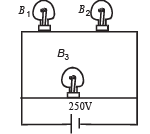Solution: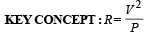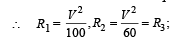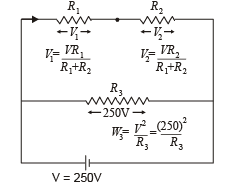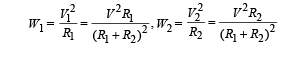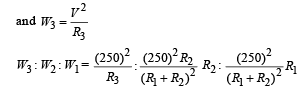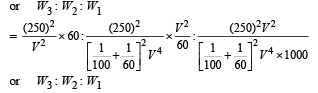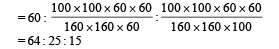QUESTION: 14

Express which of the following set ups can be used to verify Ohm’s law?

Solution:

In ohm's law, we check V = IR where I is the current flowing through a resistor and V is the potential difference across that resistor. Only option (a) fits the above criteria.
NOTE : Remember that ammeter is connected in series with resistance and voltmeter parallel with the net resistance.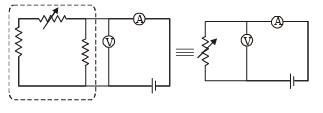QUESTION: 15

In the shown arrangement of the experiment of the meter bridge if AC corresponding to null deflection of galvanometer is x, what would be its value if the radius of the wire AB is doubled?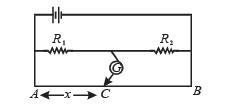Solution: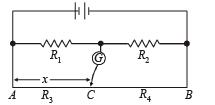At null point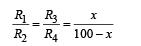If radius of the wire is doubled, then the resistance of AC will change and also the resistance of CB will change. But since R1/Rdoes not change so, R3/R4
should also not change at null point. Therefore the point C does not change.

QUESTION: 16

The three resistance of equal value are arranged in the different combinations shown below. Arrange them in increasing order of power dissipation.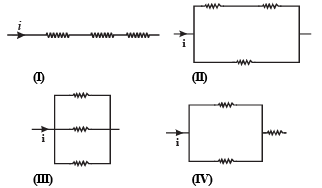Solution:

III < II < IV < I.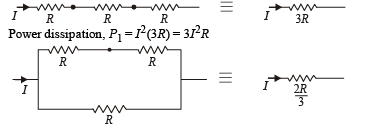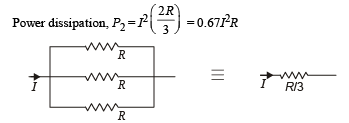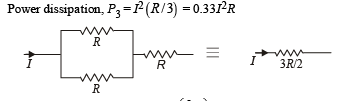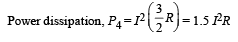QUESTION: 17

Shown in figure is a Post Office box. In order to calculate the value of external resistance, it should be connected between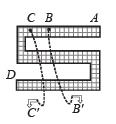Solution:

Total external resistance will be the total resistance of whole length of box. It should be connected between A and D.

QUESTION: 18

Six identical resistors are connected as shown in the figure.The equivalent resistance will be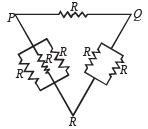Solution:

For var ious combination s equivalent r esistan ce is maximum between P and Q.

QUESTION: 19

A capacitor is charged using an exter nal batter y with a resistance x in series. The dashed line shows the variation of ln I with respect to time. If the resistance is changed to 2x, the new graph will be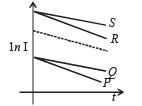Solution:

KEY CONCEPT : The current in RC circuit is given by I = I0e–t/RC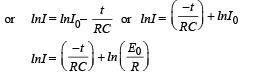On comparing with y = mx + C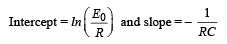When R is changed to 2R then slope increases and current becomes less. New graph is Q.

QUESTION: 20

Find out the value of current through 2Ω resistance for the given circuit.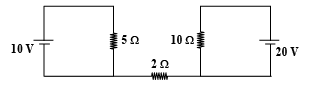Solution:

The current in 2W resistor will be zero because it is not a part of any closed loop.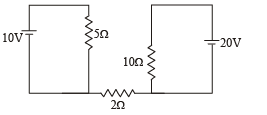QUESTION: 21

A 4 μF capacitor, a resistance of 2.5 MΩ is in series with 12 V battery. Find the time after  which the potential difference across the capacitor is 3 times the potential difference across the resistor. [Given ln(2) = 0.693]

Solution:

KEY CONCEPT : At any instant of time t during charging process, the transient current in the circuit is given by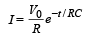∴ Potential difference across resistor R is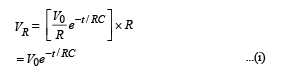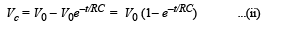∴ Potential diff. across C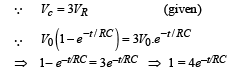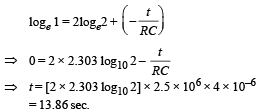QUESTION: 22

A moving coil galvanometer of resistance 100 Ω is used as an ammeter using a resistance 0.1 Ω.  The maximum deflection current in the galvanometer is 100 μA. Find the minimum current in the circuit so that the ammeter shows maximum deflection

Solution:

KEY CONCEPT :

IgG = (I – Ig) S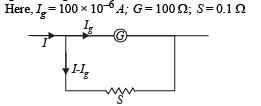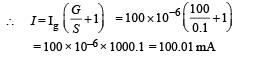QUESTION: 23

An ideal gas is filled in a closed rigid and thermally insulated container. A coil of 100 Ω resistor carrying current 1 A for 5 minutes supplies heat to the gas. The change in internal energy of the gas is

Solution:

The heat supplied under these conditions is the change in internal energy Q = ΔU
The heat supplied Q = i2RT = 1 × 1 × 100 × 5 × 60 = 30,000 J = 30 kJ

QUESTION: 24

If a steady current I is flowing through a cylindrical element ABC. Choose the correct relationship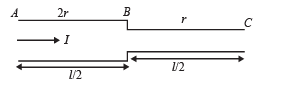Solution: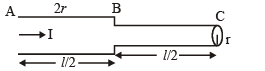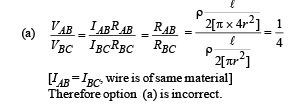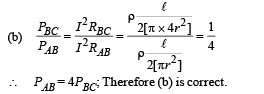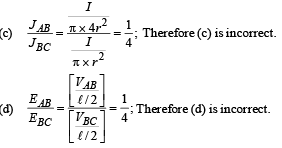QUESTION: 25

A resistance of 2Ω is connected across one gap of a metrebridge (the length of the wire is 100 cm) and an unknown resistance, greater than 2Ω, is connected across the other gap. When these resistances are interchanged, the balance point shifts by 20 cm. Neglecting any corrections, the unknown resistance is

Solution:

Given X is greater than 2Ω when the bridge is balanced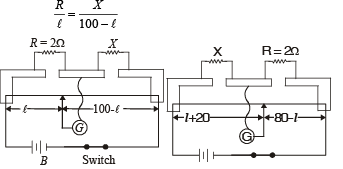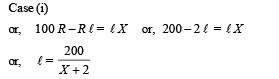When the resistances are interchanged the jockey shifts 20 cm. Therefore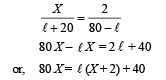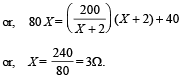QUESTION: 26

A circuit is connected as shown in the figure with the switch S open. When the switch is closed, the total amount of charge that flows from Y to X is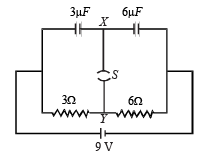Solution:

The total charge enclosed in the dotted portion when the switch S is open is zero. When the switch is closed and steady state is reached, the current I coming from the battery is 9 = I (3 + 6) ⇒ I = 1A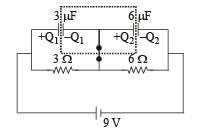∴ Potential difference across 3Ω resistance = 3V and potential difference across 6Ω resistance = 6V

∴ p.d. across 3 µF capacitor = 3V an d p.d. across 6 µF capacitor = 6V

∴ Charge on 3 µF capacitor Q1 = 3 × 3 = 9 µC Charge on 6 µF capacitor Q2 = 6 × 6 = 36 µC The total charge enclosed in the dotted portion =

∴ Charge passing the switch = 36 – 9 = 27 µC

QUESTION: 27

Figure shows three resistor configurations R1, R2 and R3 connected to 3V battery. If the power dissipated by the configuration R1, R2 and R3, is P1, P2 and P3, respectively, then –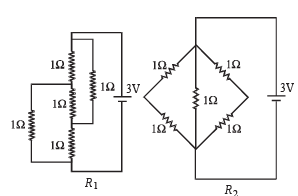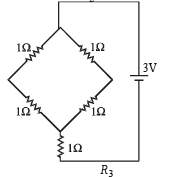Solution:

We know that
P=We know that
P=V2/R
For constant value of potential difference (V) we have
For constant value of potential difference (V) we have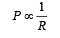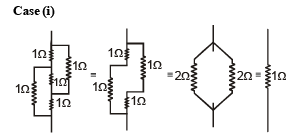This is a case of balanced Wheatstone bridge R1 = 1Ω Case (ii)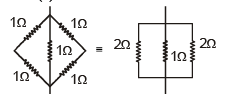Clearly the equivalent resistance (R2) will be less than 1Ω.
Case (iii)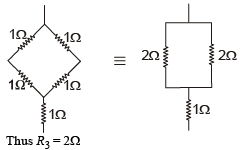Since, R2 < R1 < R3

∴ P2 > P1 > P3

QUESTION: 28

Incandescent bulbs are designed by keeping in mind that the resistance of their filament increases with the increase in temperature. If at room temperature, 100 W, 60 W and 40 W bulbs have filament resistances R100, R60 and R40, respectively, the relation between these resistances is

Solution:

We know that P=V2/R

For a given potential difference at a particular temperature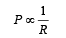It is given that the powers of the bulbs are in the order

100W > 60 W > 40W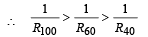QUESTION: 29

To verify Ohm’s law, a student is provided with a test resistor RT, a high resistance R1, a small resistance R2, two identical galvanometers G1 and G2, and a variable voltage source V. The correct circuit to carry out the experiment is

Solution:

c) The following points should be considered while making the circuit :

(i) An ammeter is made by connecting a low resistance R2 in parallel with the galvanometer G2.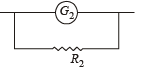(ii) A voltmeter is made by connecting a high resistance R1 in series with the galvanometer G1.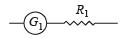(iii) Voltmeter is connected in parallel with the test resistor RT.

iv) Ammeter is connected in series with the test resistor RT.
(v) A variable voltage source V is connected in series with the test resistor RT.

QUESTION: 30

Consider a thin square sheet of side L and thickness t, made of a material of resistivity ρ. The resistance between two opposite faces, shown by the shaded areas in the figure is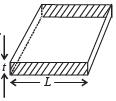Solution:

We know that R=ρ l/a

Where l is the length of the conductor through which the current flows and a is the area of cross section.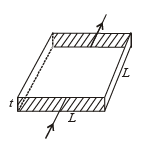Here l = L and a = L × t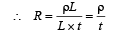∴ R is independent of L

QUESTION: 31

A meter bridge is set up as shown, to determine an unknown resistance ‘X’ using a standard 10 ohm resistor. The galvanometer shows null point when tapping-key is at 52 cm mark. The end-corrections are 1 cm and 2 cm respectively for the ends A and B. The determined value of ‘X’ is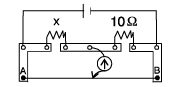Solution:

At Null point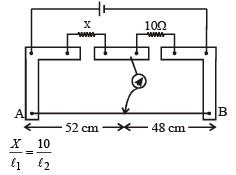Here l1 = 52 + End correction = 52 + 1 = 53 cm

l2 = 48 + End correction = 48 + 2 = 50 cm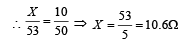QUESTION: 32

During an experiment with a metre bridge, the galvanometer shows a null point when the jockey is pressed at 40.0 cm using a standard resistance of 90 Ω, as shown in the figure.  The least count of the scale used in the metre bridge is 1mm. The unknown resistance isSolution:

In case of a meter bridge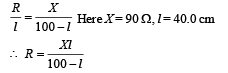For finding the value of R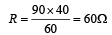For finding the value of ΔR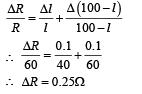Therefore, R = (60 ± 0.25)Ω

QUESTION: 33

An infinite line charge of uniform electric charge density λ lies along the axis of an electrically conducting infinite cylindrical shell of radius R. At time t = 0, the space inside the cylinder is filled with a material of permittivity ε and electrical conductivity σ. The electrical conduction in the material follows Ohm's law. Which one of the following graphs best describes the subsequent variation of the magnitude of current density j(t) at any point in the material?

Solution: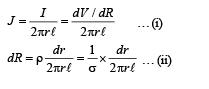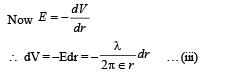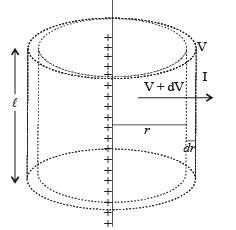From (i), (ii), and (iii)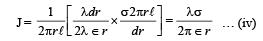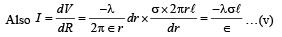Here negative sign signifies that the current is decreasing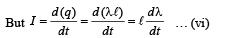From (v) and (vi)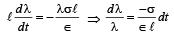On integrating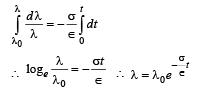Substituting this value in (iv) we get